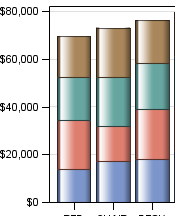## Proc template/proc sgrender stacked bar chart.

I am trying to create a stacked bar chart with proc template and sgrender

but the variable that i want on the top part of the stack will only work on the bottom:

i have

barchart x=a y=b

barchart x=a y= c

I want it to be c on top but it only works with b on top.

1 ACCEPTED SOLUTION

Accepted Solutions

## Re: Proc template/proc sgrender stacked bar chart.

I was able to figure it out where I could have total of c+b as the first y variable, and then b on the bottom as the second variable.

in proc template

xaxis

yaxia

barchart x= y=total

barchart x= y=bottomamount

16 REPLIES 16

## Re: Proc template/proc sgrender stacked bar chart.

show your entire code and some example data to show the result you get and hopefully some way to show what you want to get (image is fine PNG, GIF what ever, Excel not so fine)

Order of statements in the template, order of data and data values may have some pretty complex interactions.

## Re: Proc template/proc sgrender stacked bar chart.

Proc template;
define statgraph Graph;
begingraph;
layout overlay/
xaxisopts=(name = 'bin_label' label='a' labelattrs=(size=12pt weight=bold)tickvalueattrs=(size=10))
yaxisopts=(name = 'b' label='b' linearopts=(tickvalueformat=comma8.) labelattrs=(size=12pt weight=bold)tickvalueattrs=(size=10))
y2axisopts=(name='pct_row' label='c' labelattrs=(size=12pt weight=bold)tickvalueattrs=(size=10));
barchart x= a. y=b/ fillattrs=(color=lightgreen) name='data1'  barwidth=0.65 barlabelattrs=(size=10pt);
barchart x= a y=c / fillattrs=(color=gold) name='data2'' barwidth=0.65 barlabelattrs=(size=10pt );

## Re: Proc template/proc sgrender stacked bar chart.

data is?

Instructions here: https://communities.sas.com/t5/SAS-Communities-Library/How-to-create-a-data-step-version-of-your-dat... will show how to turn an existing SAS data set into data step code that can be pasted into a forum code box using the {i} icon or attached as text to show exactly what you have and that we can test code against.

And the sgrender code used is?Jay54
Meteorite | Level 14

## Re: Proc template/proc sgrender stacked bar chart.

While you say "Stacked" bar chart, you are showing code that will generate "Overlaid" bars.  Since y=c is specified later, it will be drawn later, overlaid on the y=b bar.  The bar charts are drawn in the order you specify them.  If you want y=b layered on top, put it later in the code.

This bar chart is not "Stacked".  To get a stacked bar chart, use the GROUP option.  The group values will be stacked by default.  To get side-by-side bars, use GROUPDISPLAY=CLUSTER.

## Re: Proc template/proc sgrender stacked bar chart.

Thanks, but the variables are really able to be grouped. they are two separate columns

## Re: Proc template/proc sgrender stacked bar chart.

IMO it's easier to restructure data than to modify a template.

@emcw wrote:

Thanks, but the variables are really able to be grouped. they are two separate columns

## Re: Proc template/proc sgrender stacked bar chart.

Are you trying to stack C on top of B in the same bar?

## Re: Proc template/proc sgrender stacked bar chart.

Yes that's right. Id prefer not to share the data

## Re: Proc template/proc sgrender stacked bar chart.

@emcw wrote:

Yes that's right. Id prefer not to share the data

MAKE FAKE DATA. We don't need real data, just data that looks like what your is and can mimic it. We all have confidential information.Jay54
Meteorite | Level 14

## Re: Proc template/proc sgrender stacked bar chart.

Unlike Excel, two separate columns cannot be stacked in GTL or SGPLOT. You can place them side by side using DISCRETEOFFSET. To stack the values, you have to change the structure, and convert the 2-column data into a group and response data.    You can use PROC TRANSPOSE, or just Data Step as below.

data b;

set a;

keep a Group Value;

Group='B'; Value=b; output;

Group='C'; Value=c; output;

run;

## Re: Proc template/proc sgrender stacked bar chart.

The group won't work because I need the count.

for example

c=4,9,5,8

b=2,1,3,2

with b on top, the one with lower value.Jay54
Meteorite | Level 14

## Re: Proc template/proc sgrender stacked bar chart.

It would be easier and faster to help if you include a picture of what you want, and your code with a (fake) data set (in code).  Which release of SAS are you using?

## Re: Proc template/proc sgrender stacked bar chart.

somthing like this.

with c=4,5,7,8

b=2,1,3,1

i was thinking i could have totalbc as a new variable, then group=c, group=b but the grouping isn't workingJay54
Meteorite | Level 14

## Re: Proc template/proc sgrender stacked bar chart.

See this article on how to create such a graph.  The data needs to be in "Grouped" form as in SASHELP.PRDSALE.

Discussion stats
• 16 replies
• 3356 views
• 0 likes
• 4 in conversation